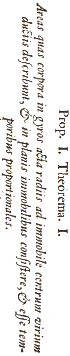Astronomy 1 Dynamical Astronomy Prof. G. Woan Room 222, Kelvin Building.  <<<<<<< .mine 10 lectures, starting Monday 19 September 2017 ||||||| .r461 9 lectures, starting Monday 26 September 2016 ======= 10 lectures, starting Tuesday 19th September 2017 >>>>>>> .r474 This course introduces the student to Newtonian Gravitation, and its application to simple two body problems. We will cover ideas of force, energy, momentum, and angular momentum as applied to the motion of a body in a gravitational field, and explore how the parameters of the orbit cam be deduced from observations of the motion.

You will need a password for off-site access to the notes.
See the Moodle link to this site.

• Course details and lecture notes
• Recommended books
•# Course details

Copies of lecture notes appear here for reference.  You will find the course harder if you don't create your own written version, so please do not print these notes out in quantity.

Introduction Motion under gravity; gravity as a long range force; Newton's Law of Universal Gravitation; gravitational attraction of spherical bodies; 1, 2 and n-body interactions; Kepler's Laws of planetary motion
 lecture notes: 1 2 3 4 5 6 7
Pictures: [ galaxy M83globular cluster 47 Tuc ]
Handouts:[ quick facts #1: orbital motion | force from a spherical body (not examinable) | Newton's Principia ]
Animations[Stars at the centre of the Galaxy  more, and more |  Newton's cannon  and web version | Cassini's orbits |   orbit simulations (2 and 3 body) ]

Planetary motion  Newton's laws of motion;  linear momentum; circular motion; angular velocity; centripetal acceleration; orbital period; Kepler's laws derived for circular orbits; natural units for the solar system; geostationary orbits; angular momentum
 lecture notes: 1 2 3 4 5 6 7
Animation: [geostationary orbit ]

Elliptical orbits  Conic sections (ellipse, circle, hyperbola); properties of the ellipse; aphelion and perihelion; response of orbits to an impulse; semi-latus rectum
 lecture notes: 1 2 3 4 5 6 7
Pictures: [ conic sections  |  comet Encke ]
Applets: [ comet Encke  67P]

Conservation laws Idea of conserved quantities; energy; momentum; angular momentum;  gravitational potential energy (general and at small heights); virial theorem; escape velocity; orbital velocity law derived
Applications Relationship between semi-major axis and energy; equation of the ellipse; proof of K1; relation between angular momentum and semi-latus rectum
 lecture notes: 1 2 3 4 5 6 7 8 9 10 11 12 13 14
Handouts: [ Kepler's laws from Newtonian dynamics (not examinable)]
Links: [Emmy NoetherGW150914 (orbit decays because of gravitational waves) ]

Hohmann transfer orbits As the 'most efficient' transfer orbit; example of LEO-->GEO; transfer times and delta-vees; example of Earth-->Mars transfer
 lecture notes: 1 2 3 4 5 6 7
sites: [ Mars Maven Mission (transfer orbit example)]
Picture: [Exomars transfer orbitMaven transfer orbit | Venus Express transfer orbit ]

Rockets  Gravity assist; examples of Voyager and Cassini; the rocket equation
 lecture notes: 1 2 3 4 5 6 7 8
Pictures: [ Cassini | Cassini flightpath ]
Links: [JPL Horizons  and coding examples (in Python)]

The two-body problem  Form of two-body orbits; relative motion;  reduced mass and radius vector
 lecture notes: 1 2 3 4 5 6 7 8

Handouts:[ quick facts #2: The two-body problem

Example exam questions and answers with hints and tips

Learning Objectives: On completion of this course, the student should be able to apply the basic concepts of Newtonian gravitation and motion and  use these concepts quantitatively. In particular the student will be able to carry out dynamical calculations for planetary, stellar and spacecraft orbits corresponding to the planar 1 and 2 body problems.

Books
There is no single textbook which is an essential purchase for this  module. However An Introduction to Modern Astrophysics, B W Carroll and D A Ostlie, Addison Wesley is strongly recommended, and is essential for the Astronomy 2 course It has its own website here. Its approach is in places more advanced than required for A1, however there is much useful material in it.

Astronomy - Principles and Practice, 4th Edition, A E Roy & D Clarke, IoP Publishing will also be of great help. This is also useful for positional astronomy and instrumental courses.

For wider background reading, students may find the following list useful: